Rs. 999.00 Rs. 1,999.00

Choose Quantity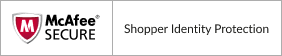Color: Black
Design Type: In-ear
Features: Noise Cancelling
Connectivity Technology: Bluetooth Wireless
Microphone: With Microphone
Country of Origin: India

View More

View Less

### You may also like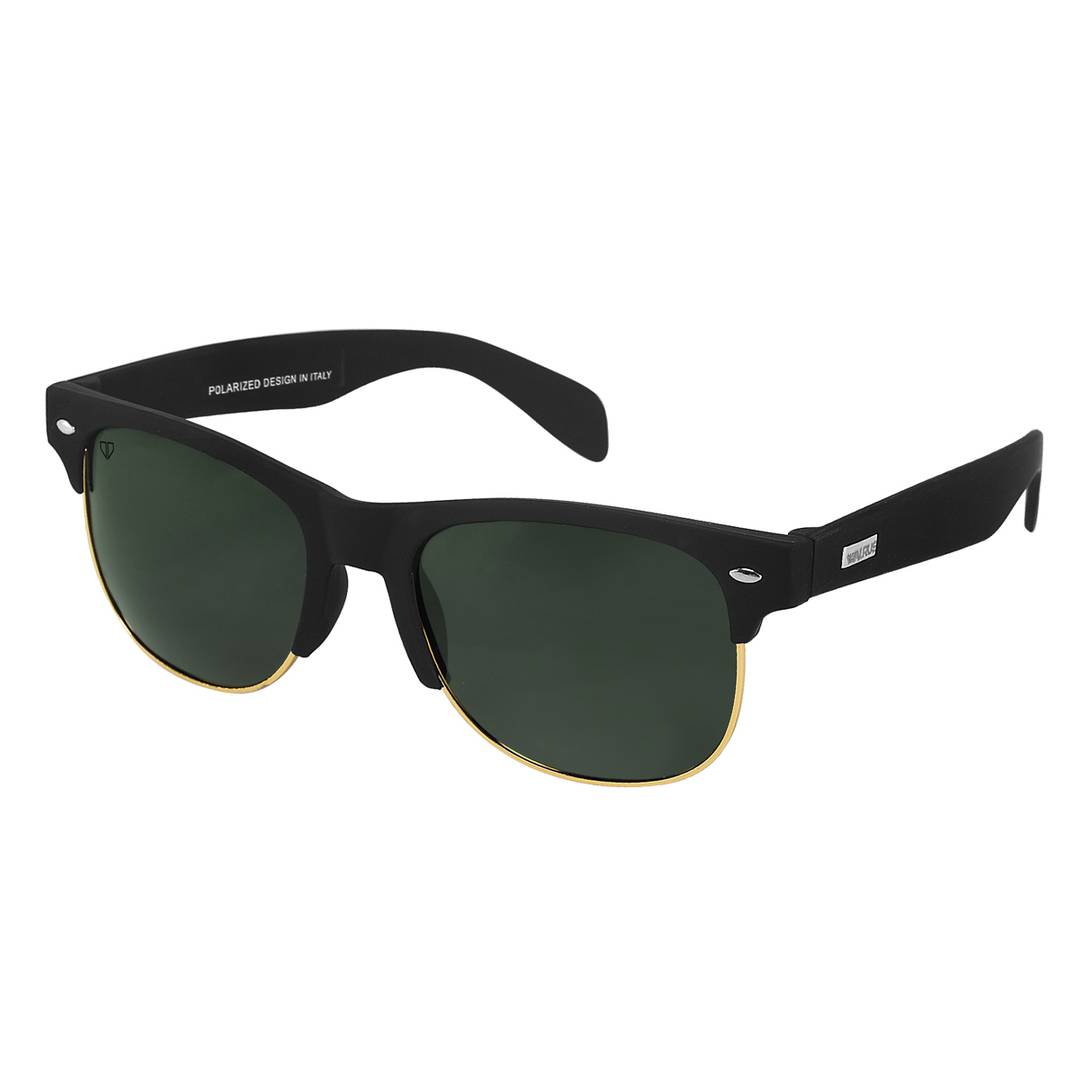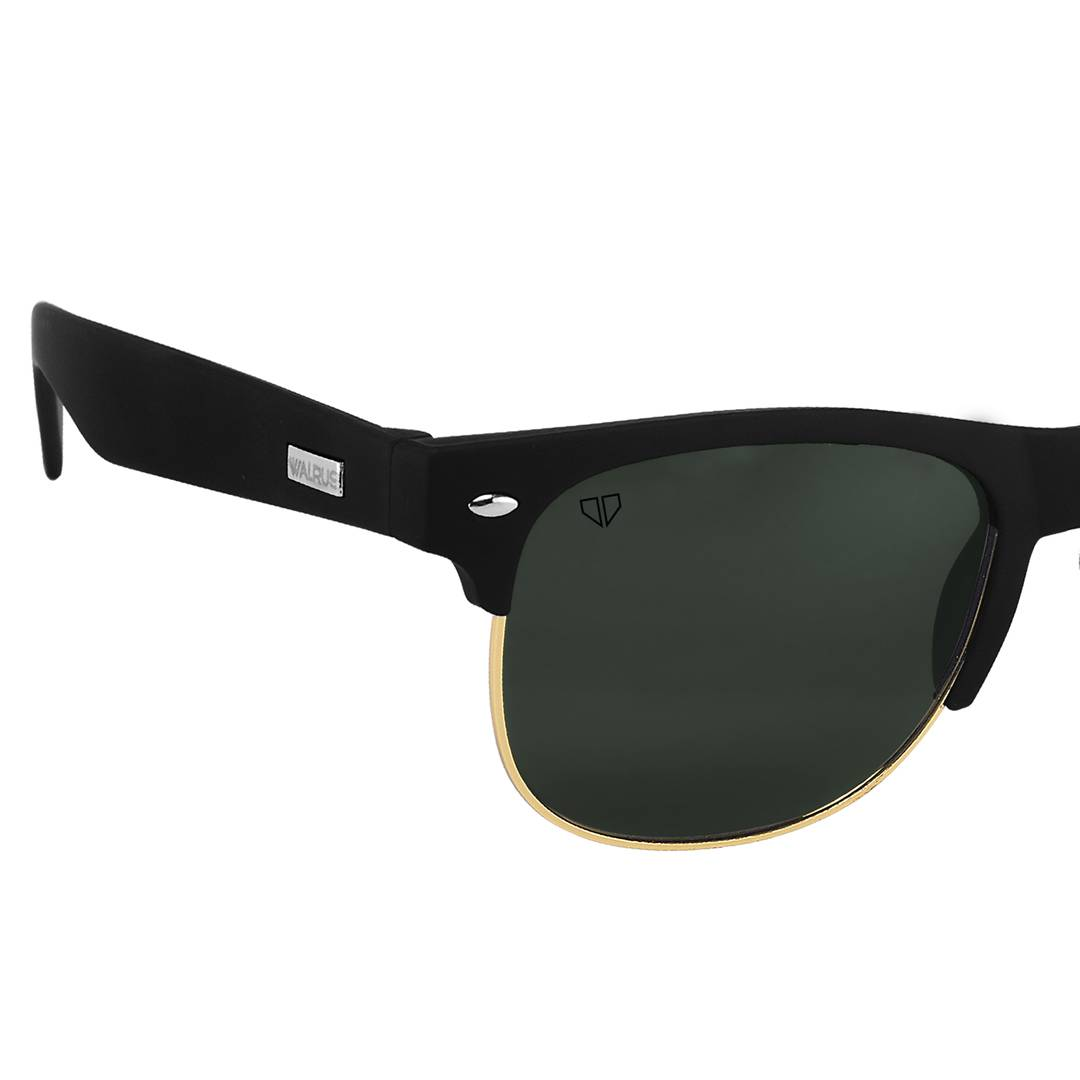Rs. 338.00
Rs. 699.00Rs. 449.00
Rs. 1,299.00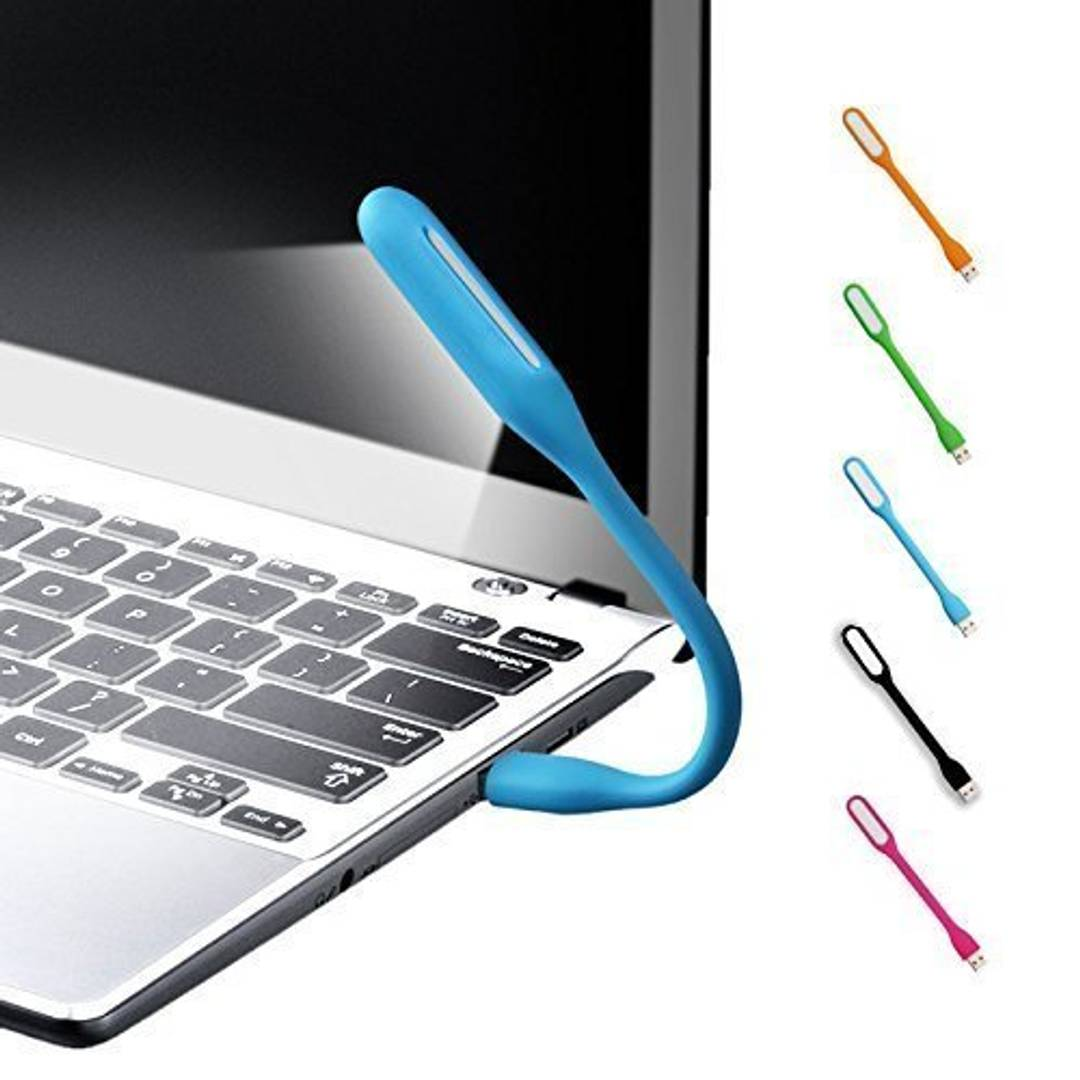Rs. 349.00
Rs. 1,199.00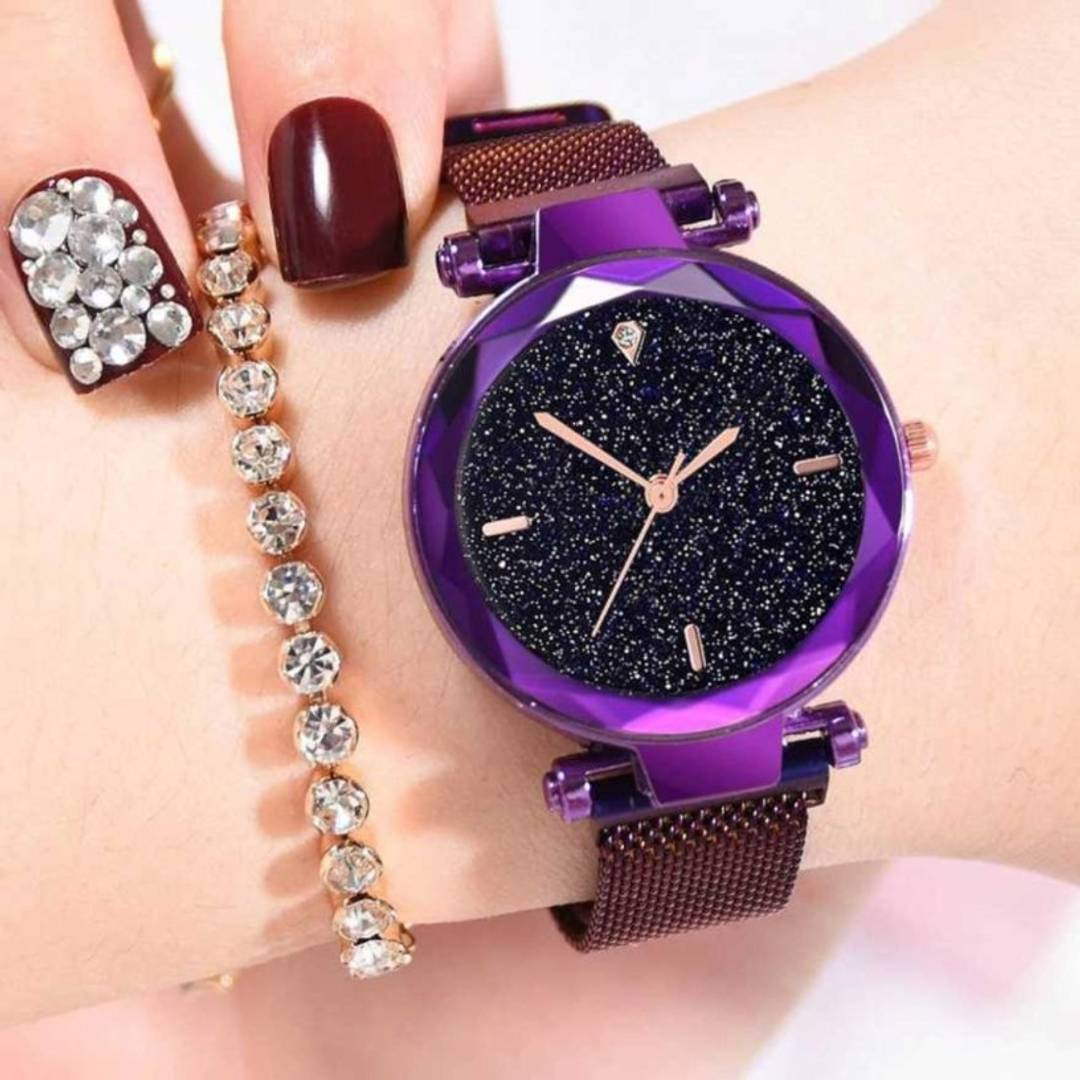Rs. 399.00
Rs. 999.00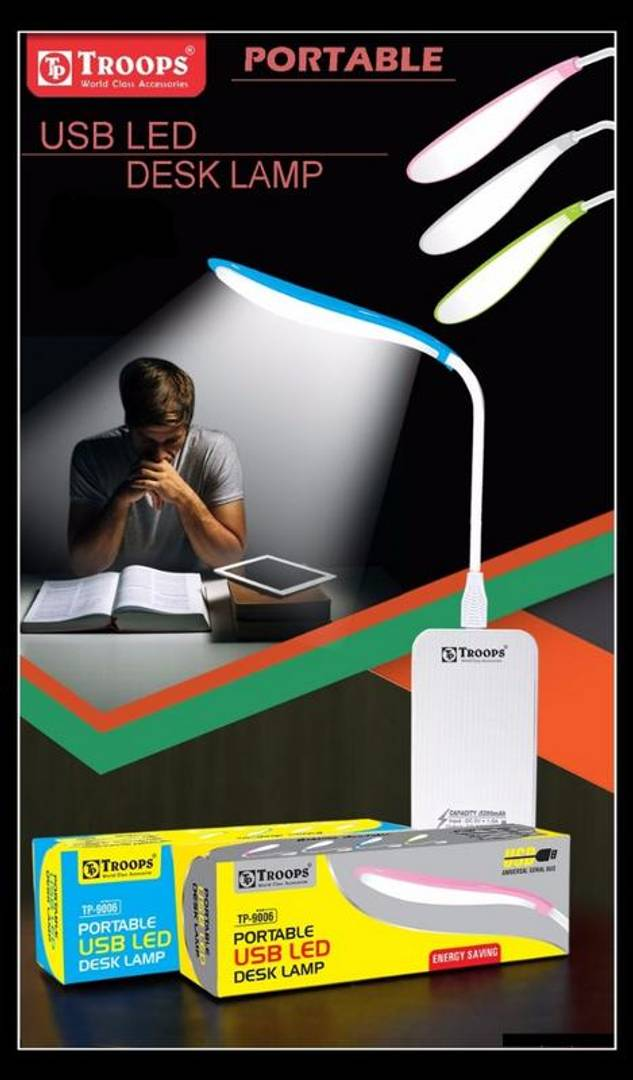Rs. 499.00
Rs. 999.00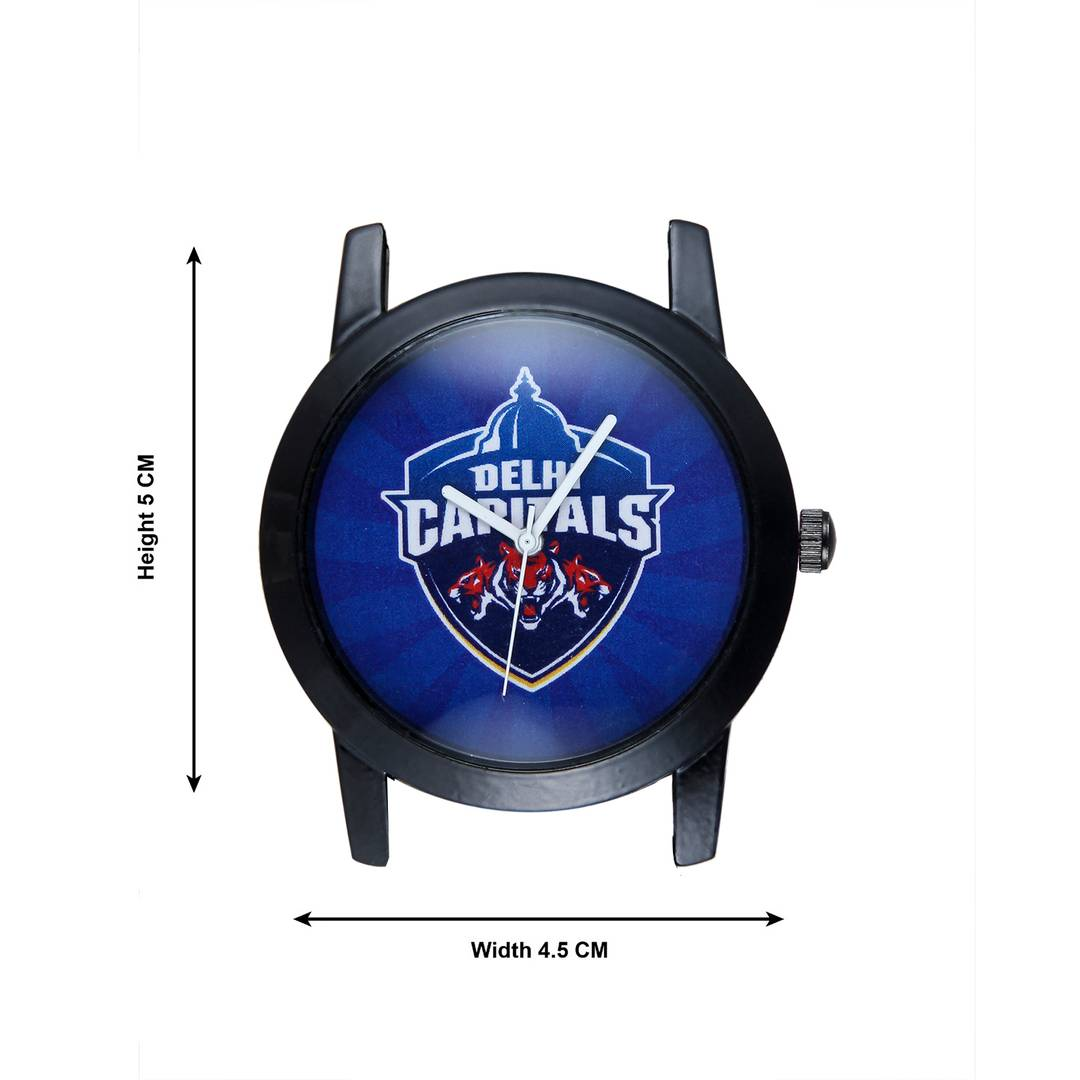Rs. 499.00
Rs. 999.00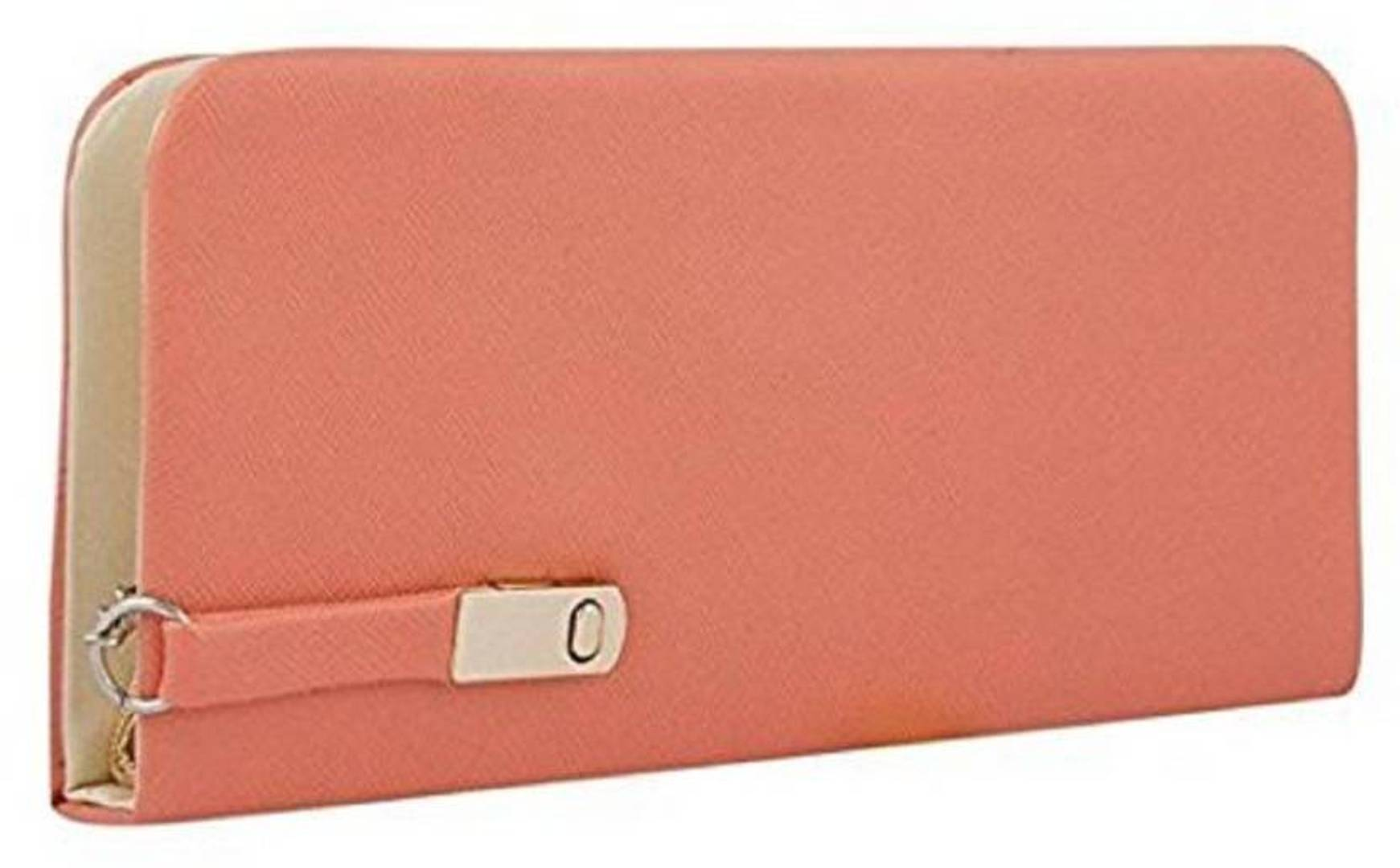Rs. 399.00
Rs. 999.00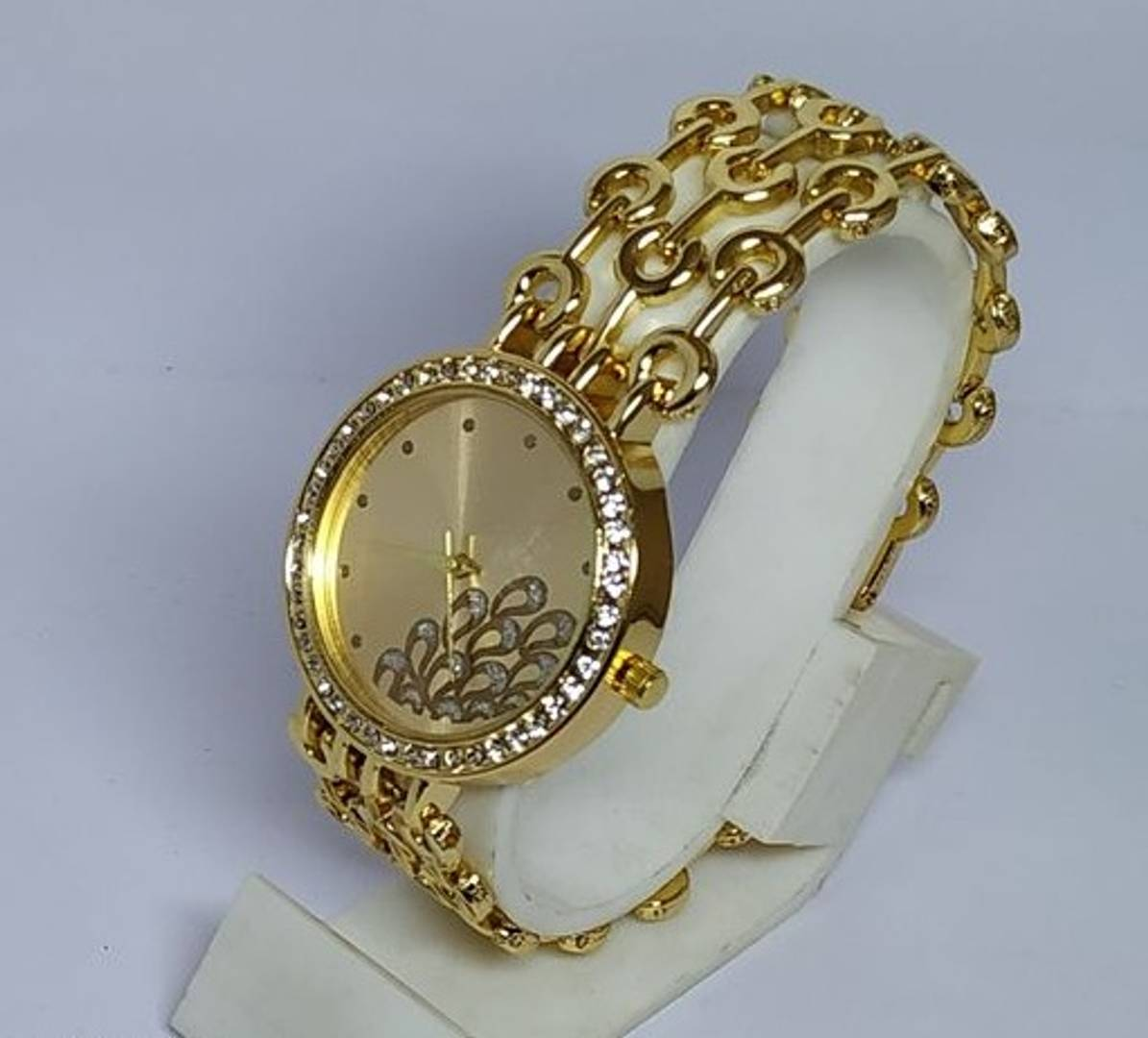Rs. 499.00
Rs. 999.00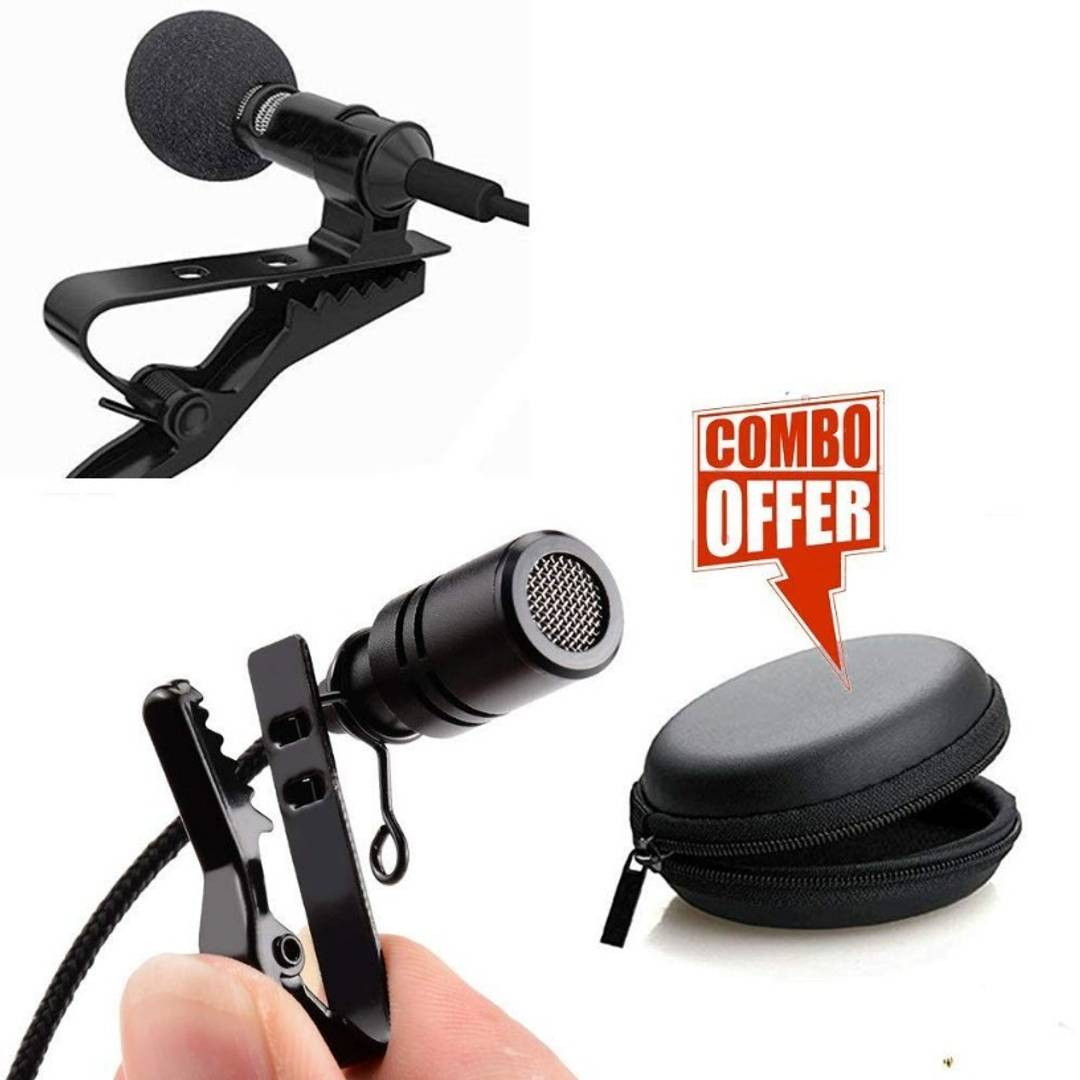Rs. 549.00
Rs. 1,399.00
Sold Out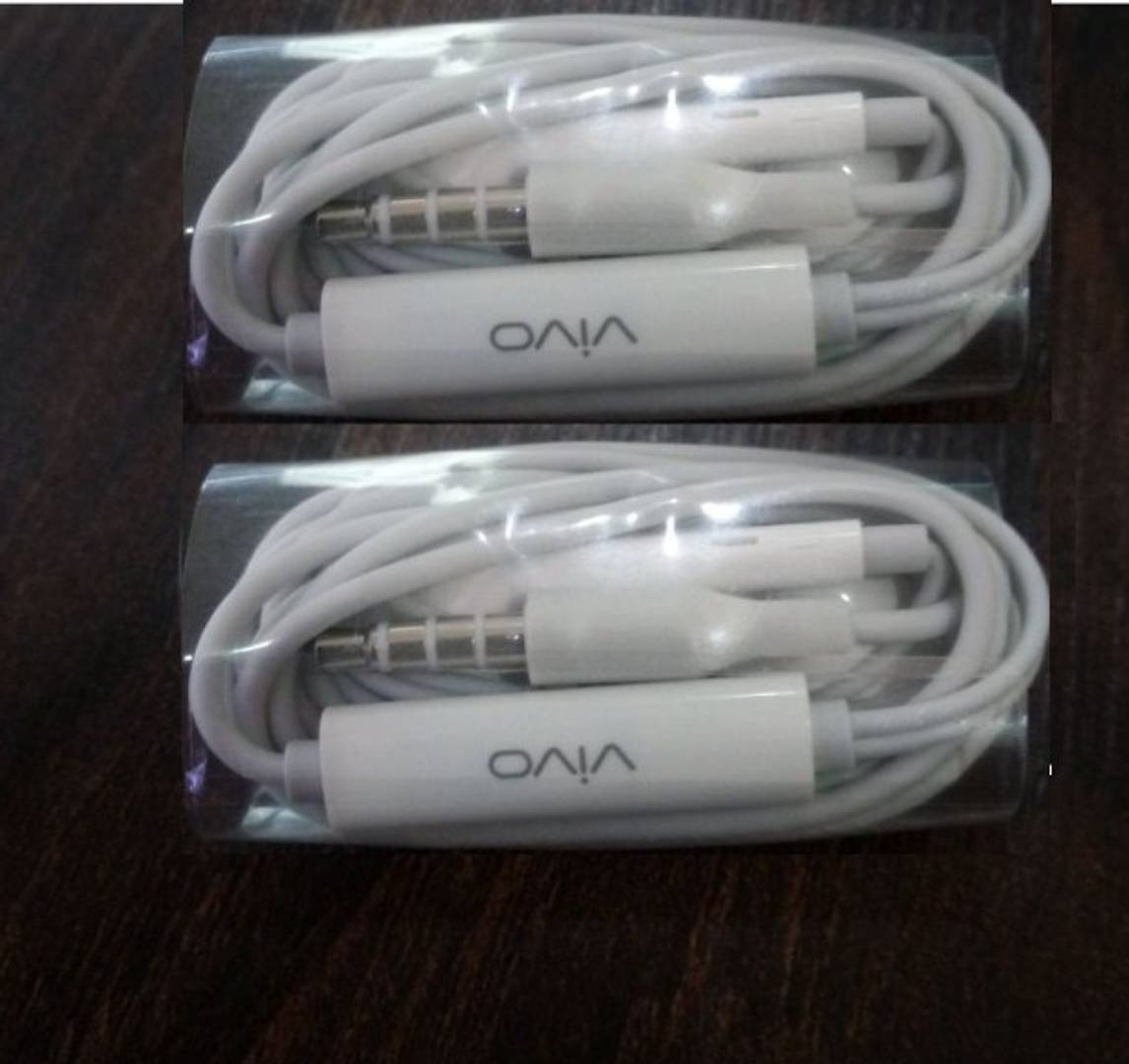Rs. 449.00
Rs. 1,299.00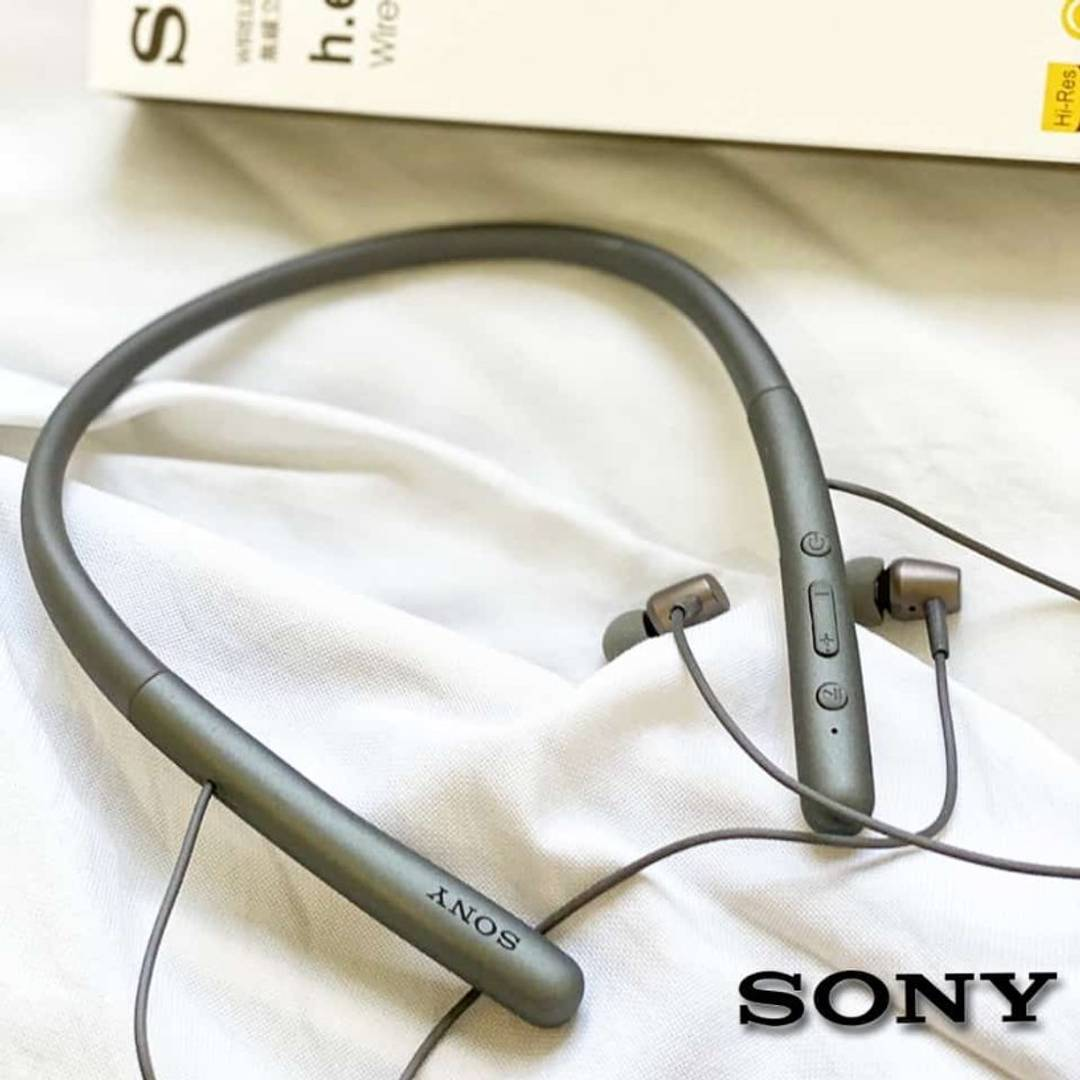Rs. 899.00
Rs. 1,899.00Rs. 649.00
Rs. 1,399.00Rs. 599.00
Rs. 1,399.00Rs. 1,499.00
Rs. 2,499.00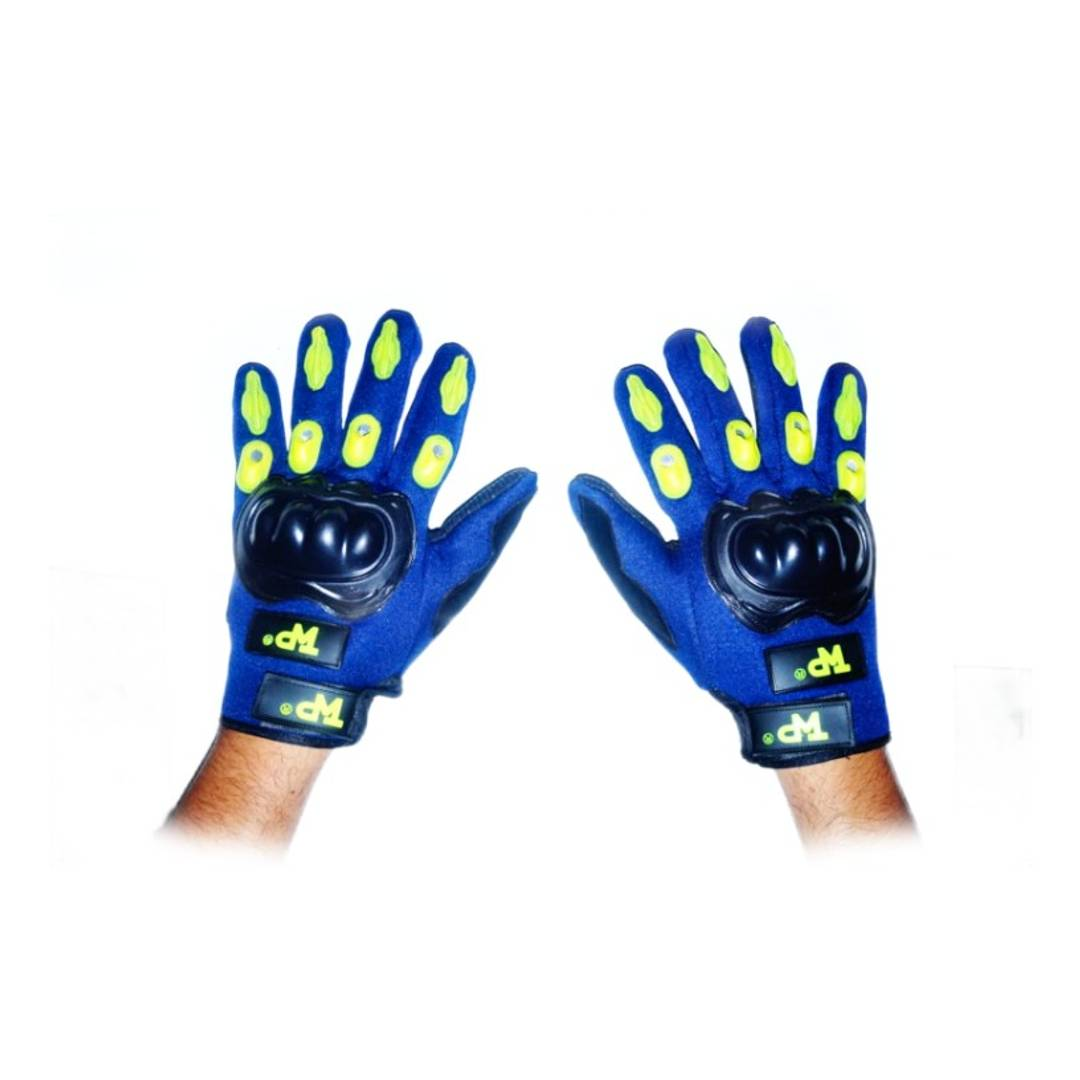Rs. 749.00
Rs. 1,299.00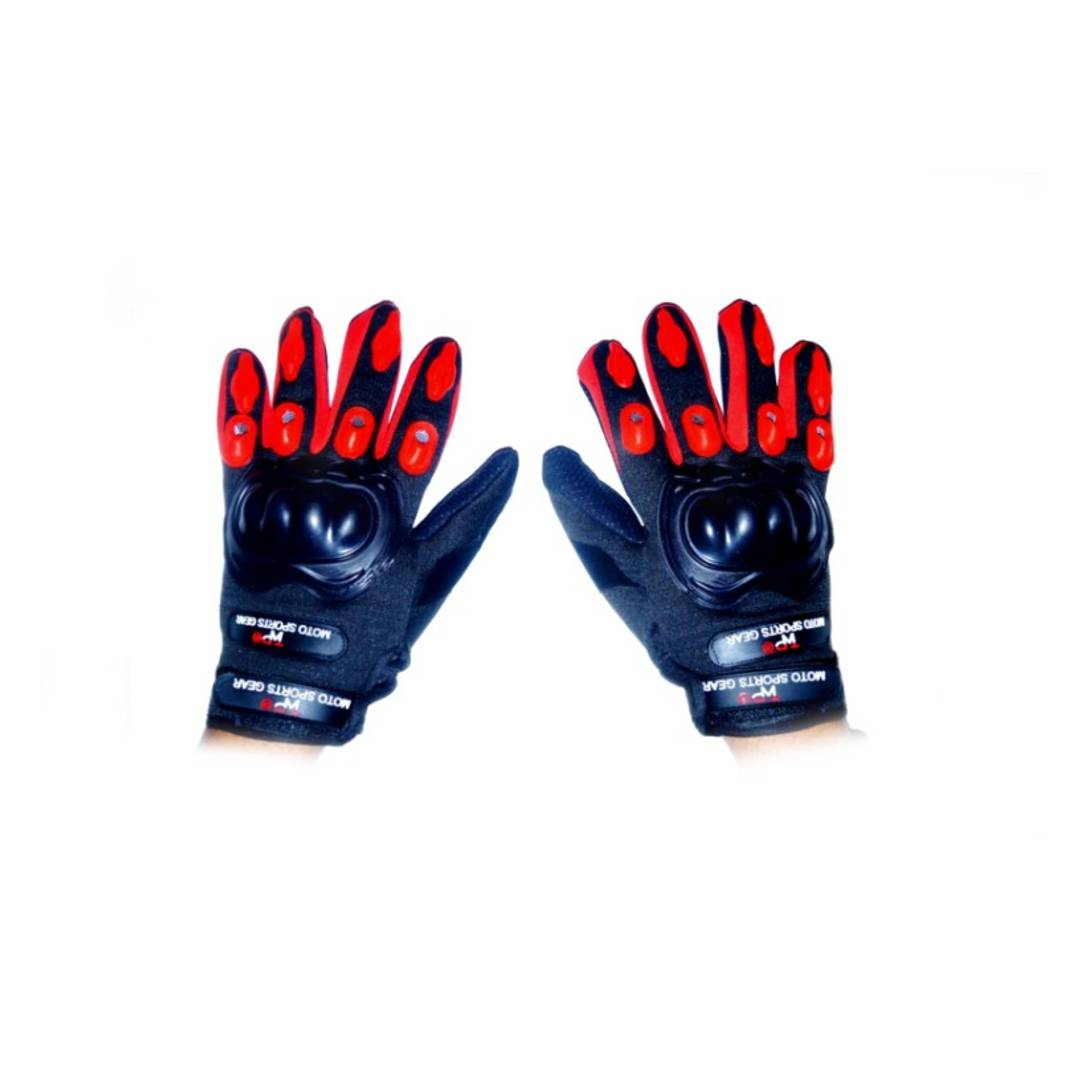Rs. 749.00
Rs. 1,299.00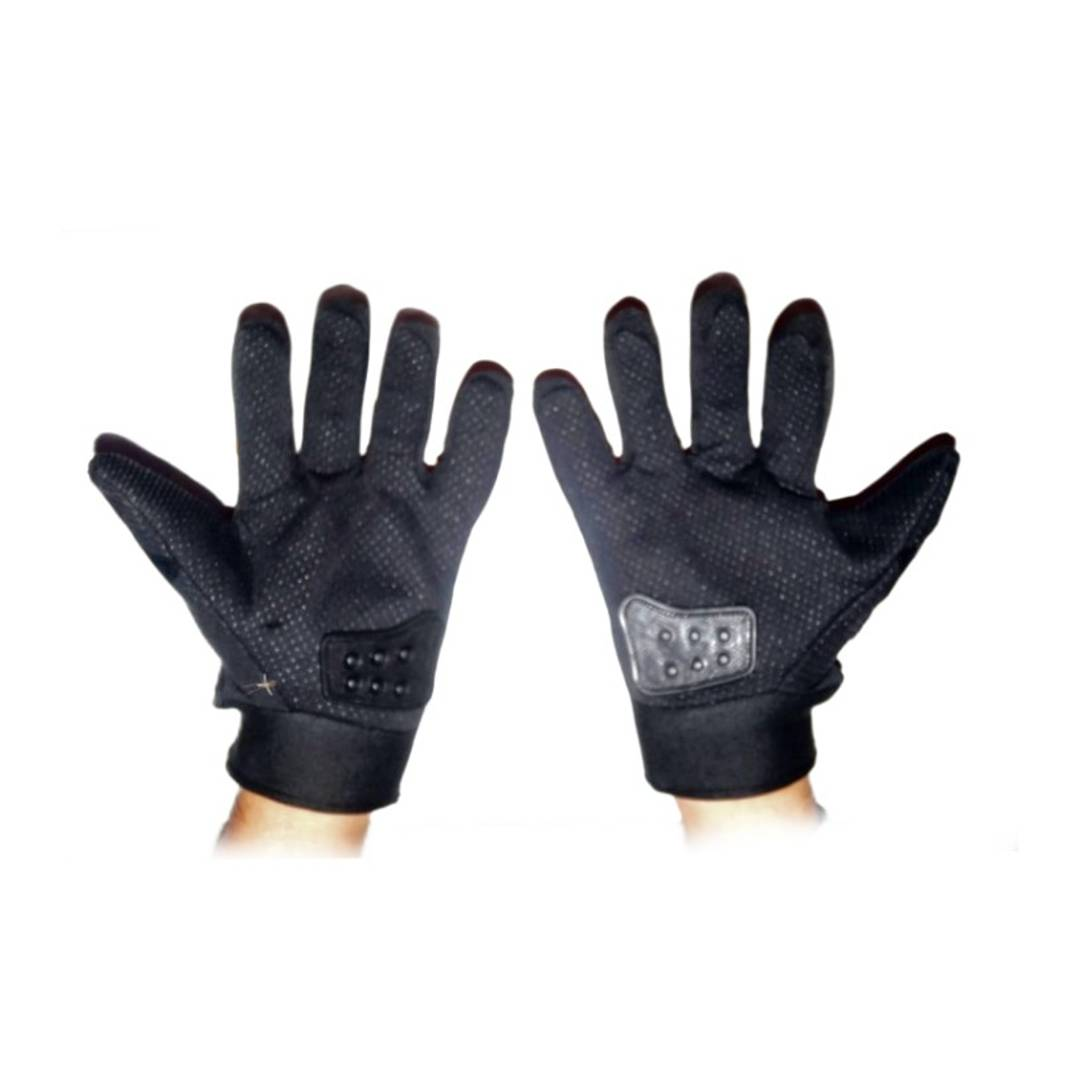Rs. 749.00
Rs. 1,299.00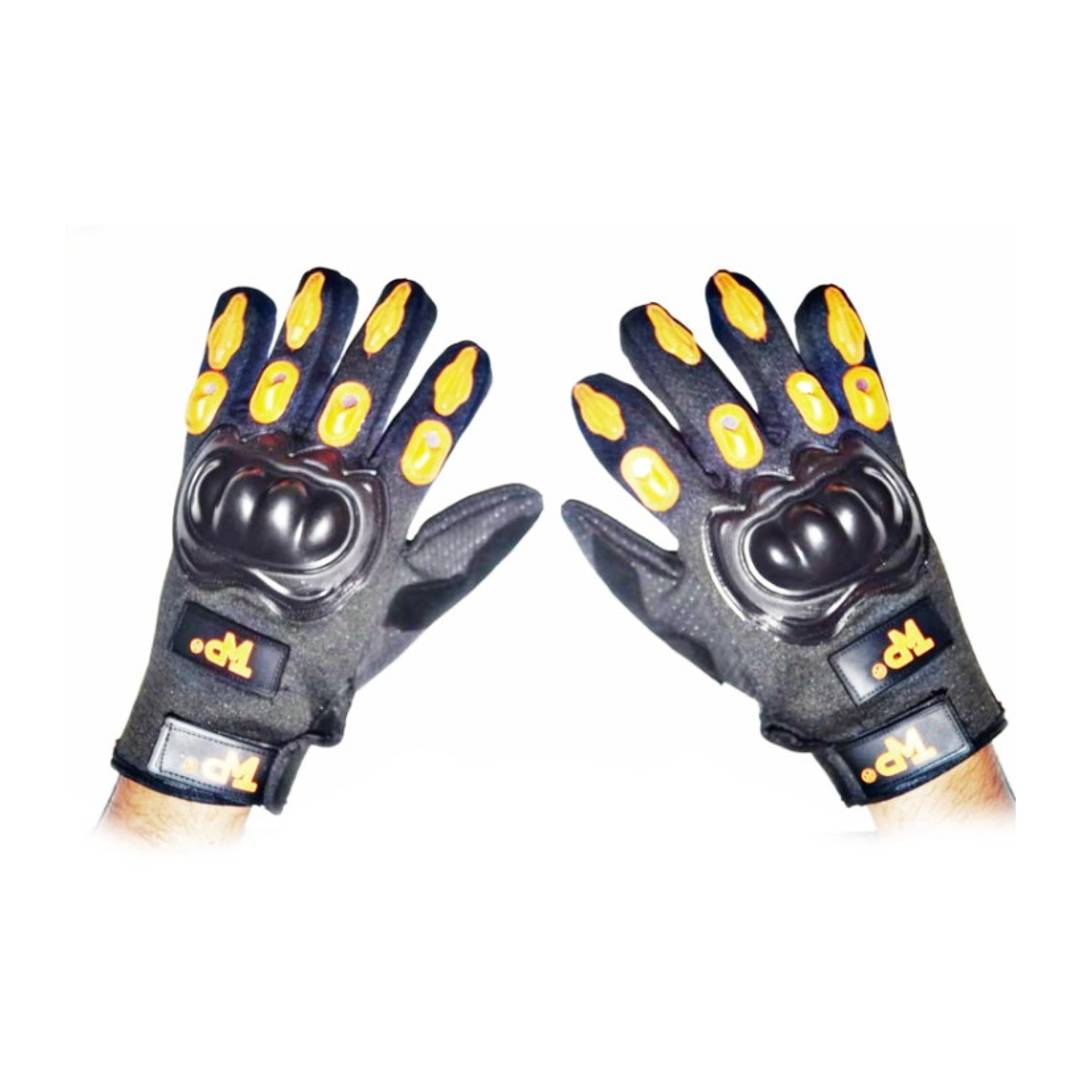Rs. 749.00
Rs. 1,299.00
Sold OutRs. 949.00
Rs. 1,499.00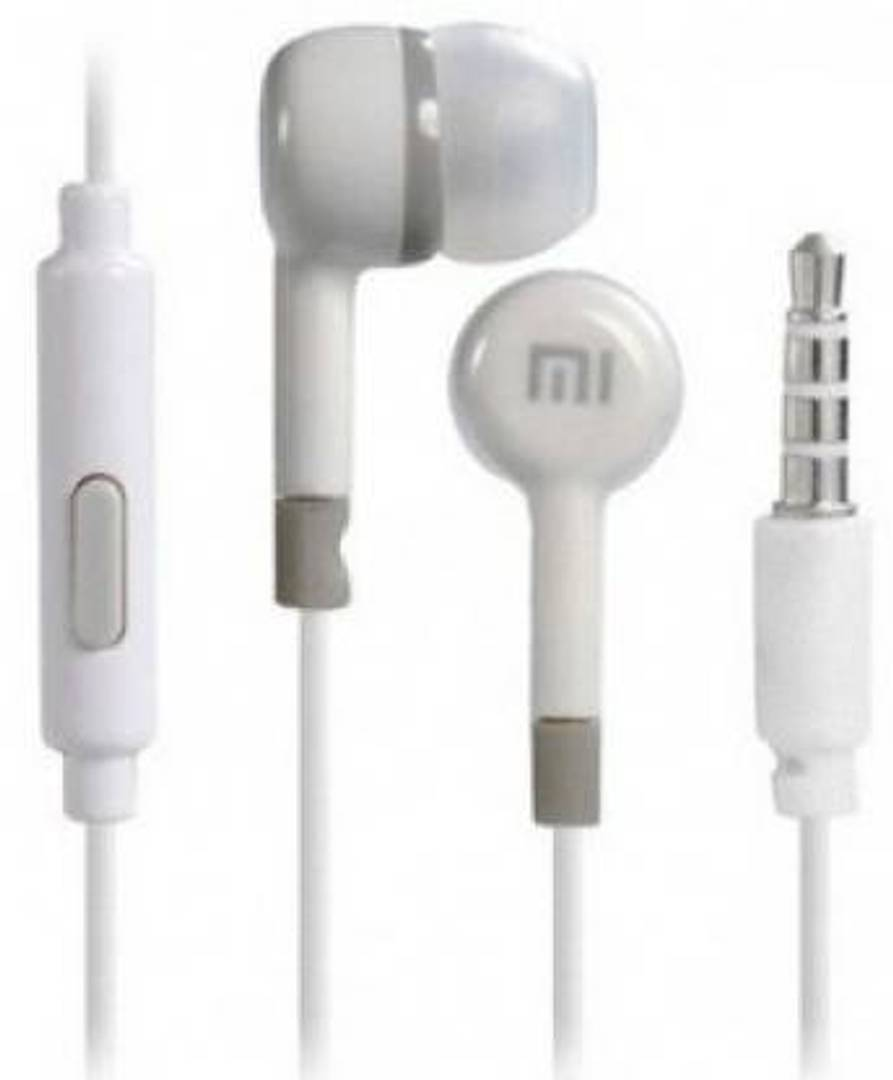Rs. 449.00
Rs. 999.00Rs. 867.00
Rs. 1,299.00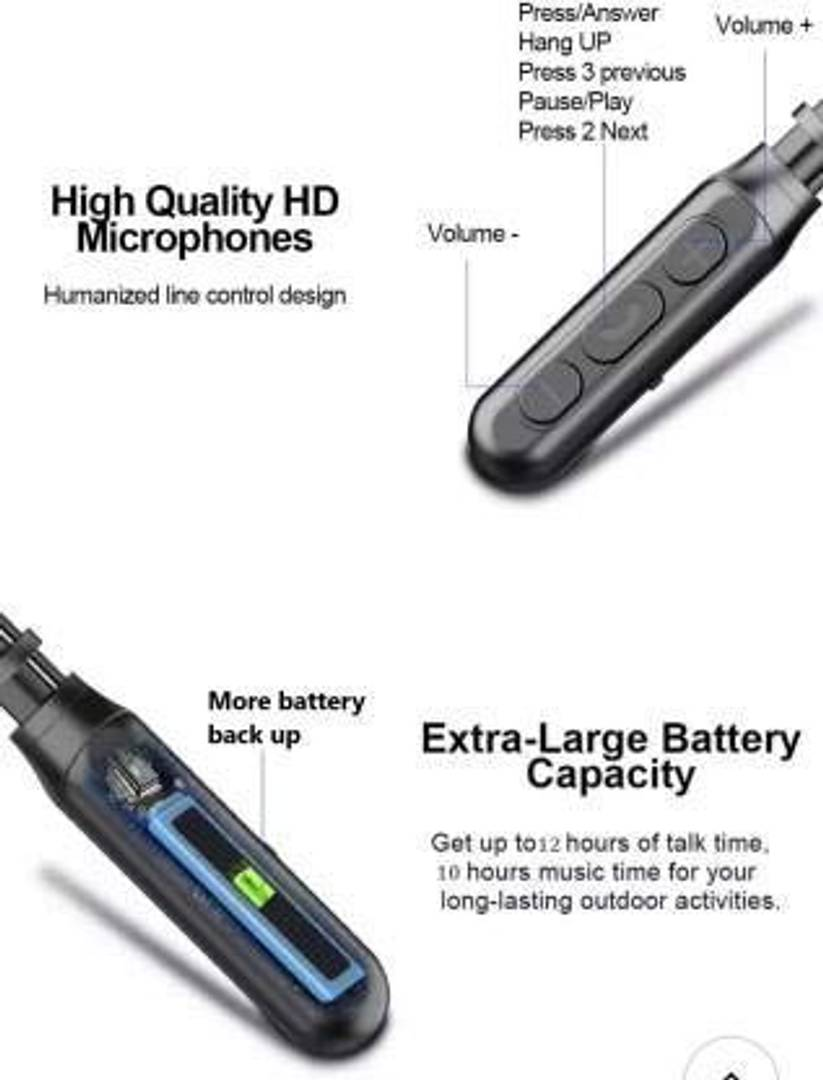Rs. 1,899.00
Rs. 2,899.00
Sold OutRs. 699.00
Rs. 1,499.00Rs. 399.00
Rs. 999.00
Sold Out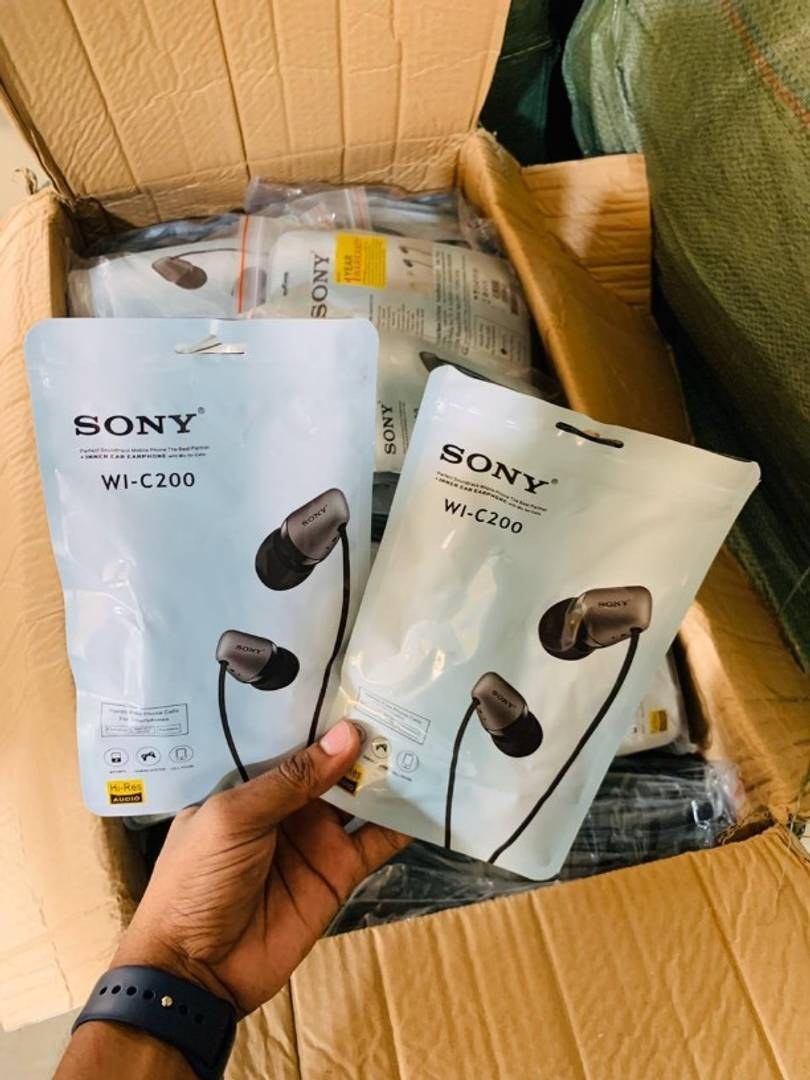Rs. 525.00
Rs. 1,499.00
Sold OutRs. 549.00
Rs. 1,499.00
Sold Out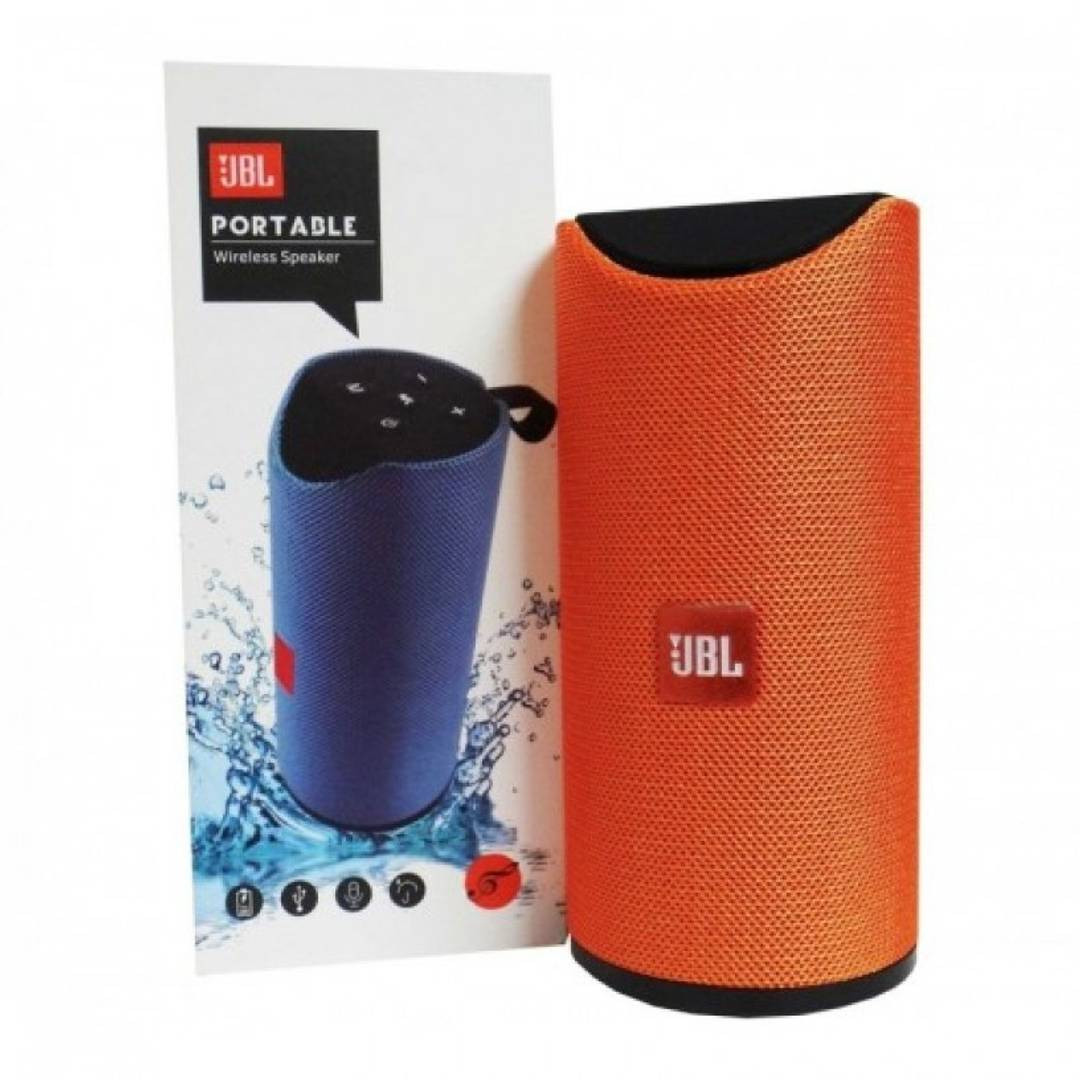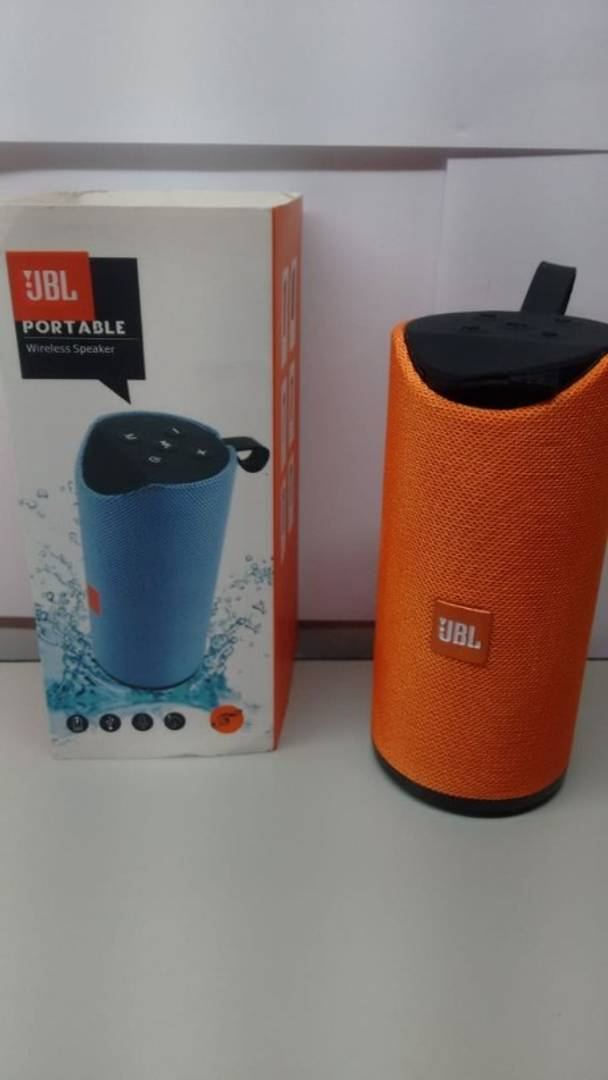Rs. 649.00
Rs. 1,599.00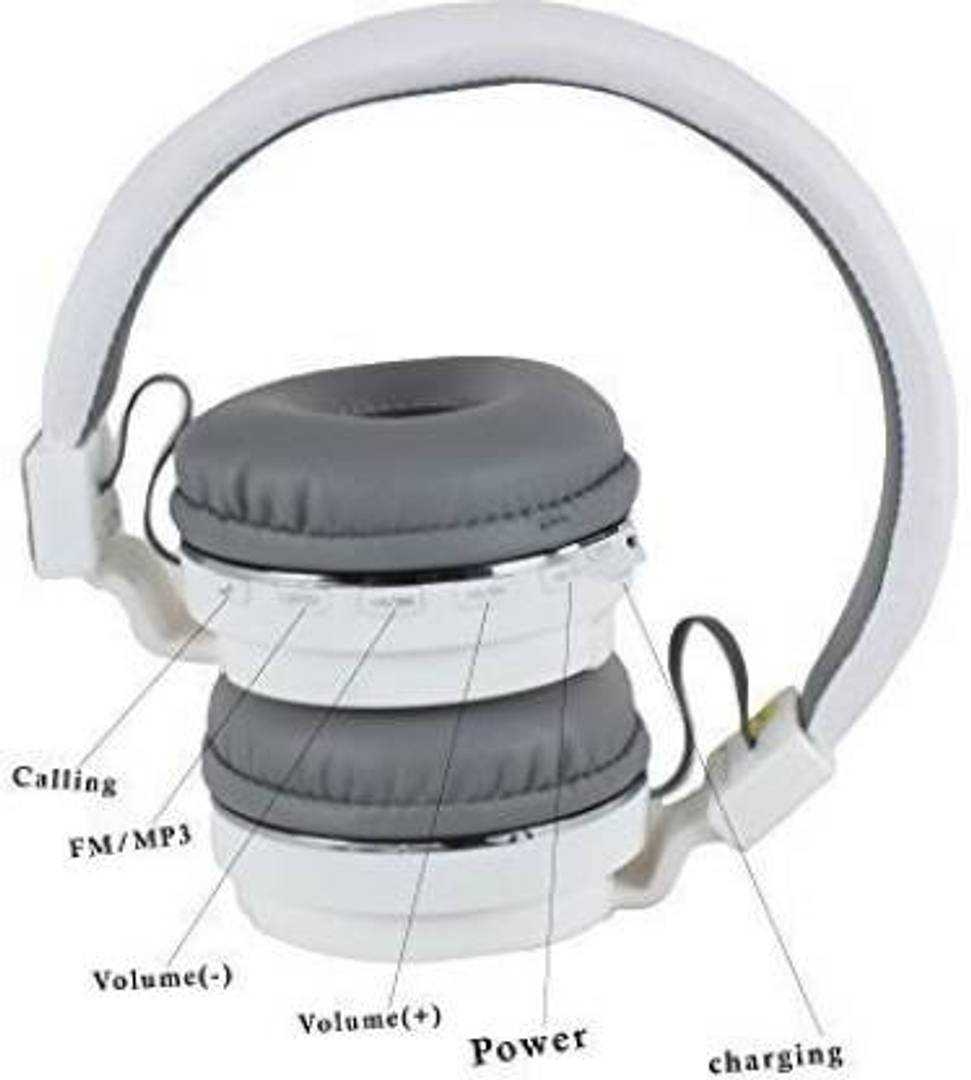Rs. 1,499.00
Rs. 2,599.00Rs. 1,499.00
Rs. 2,499.00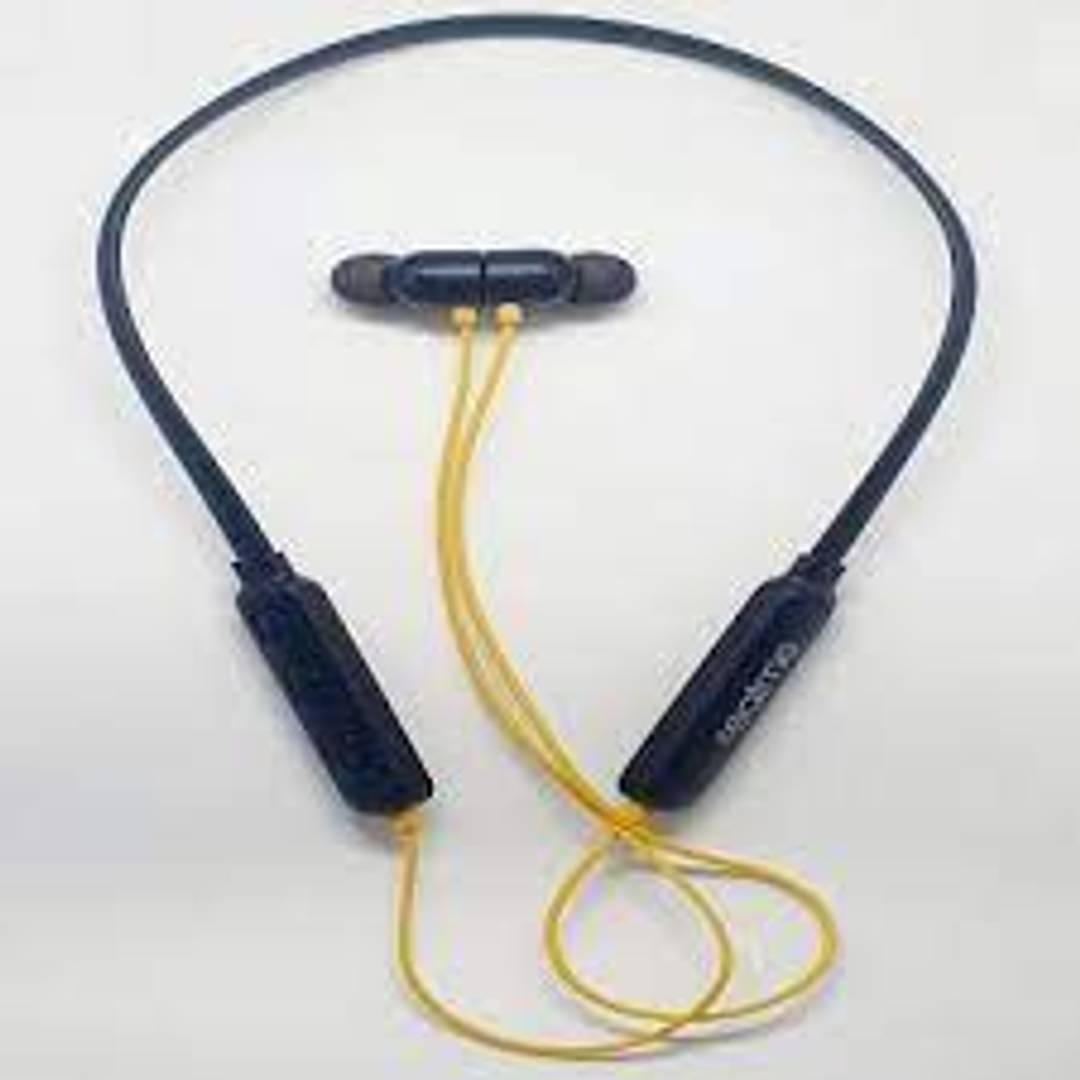Rs. 1,449.00
Rs. 2,499.00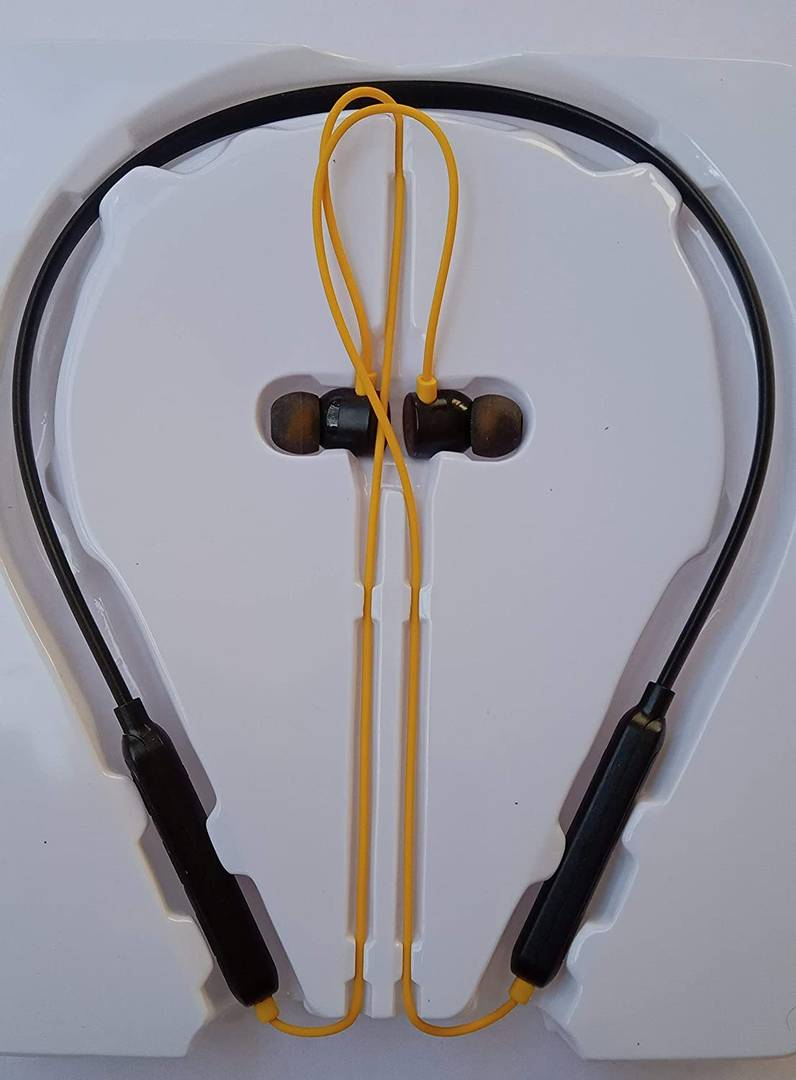Rs. 649.00
Rs. 1,499.00Rs. 899.00
Rs. 1,899.00Rs. 899.00
Rs. 1,899.00Rs. 899.00
Rs. 1,899.00
Sold OutRs. 1,199.00
Rs. 2,399.00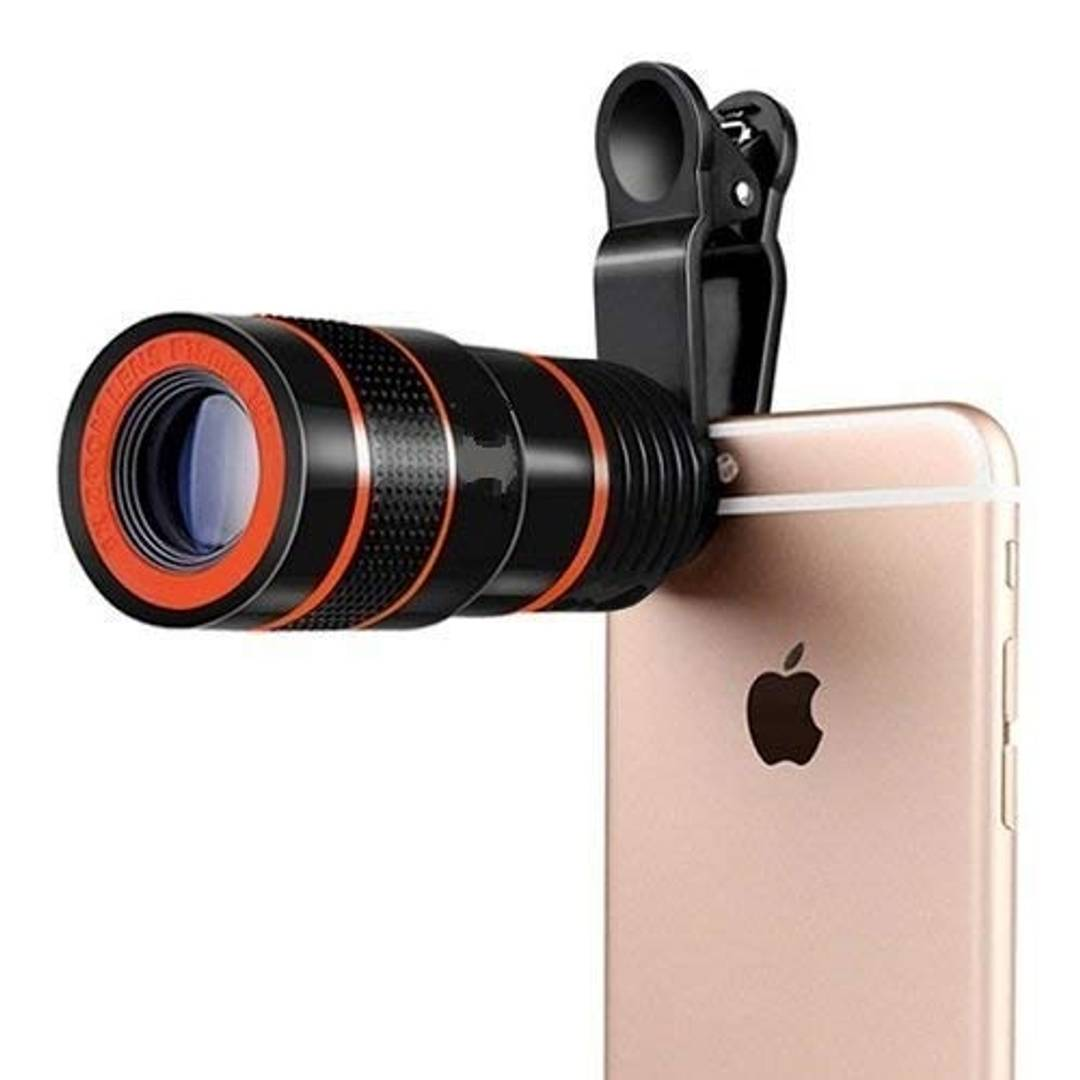Rs. 759.00
Rs. 1,499.00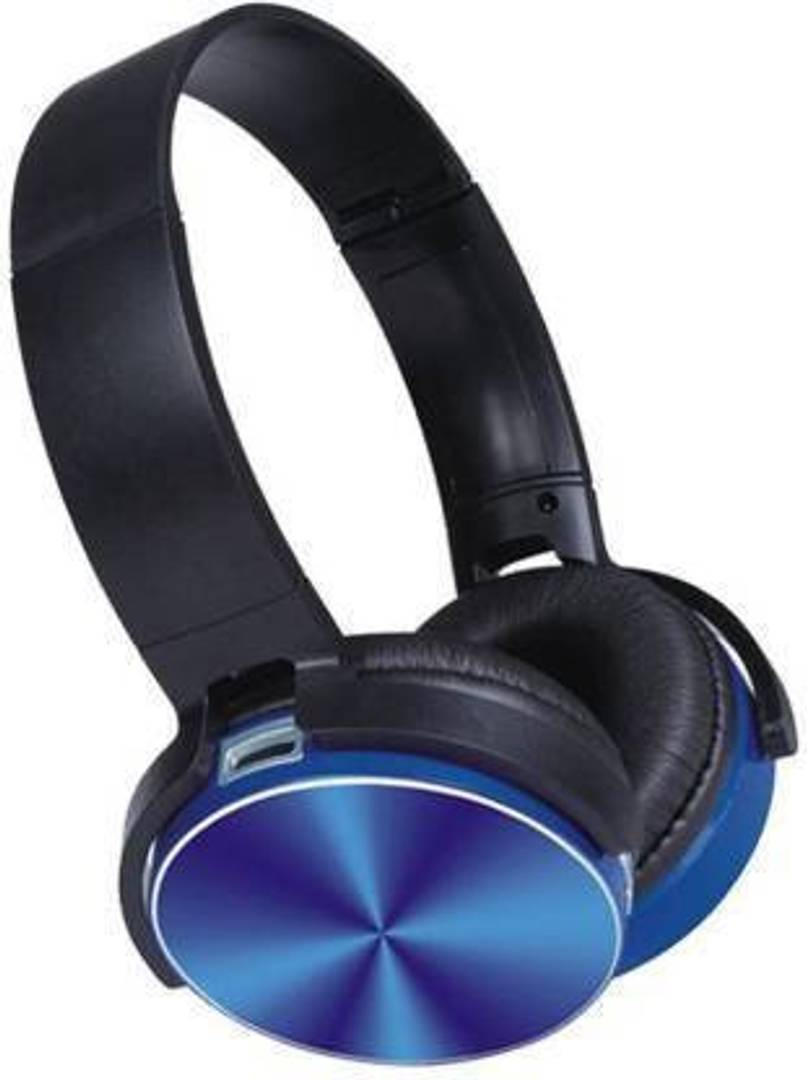Rs. 1,249.00
Rs. 2,399.00
Sold OutRs. 731.00
Rs. 1,499.00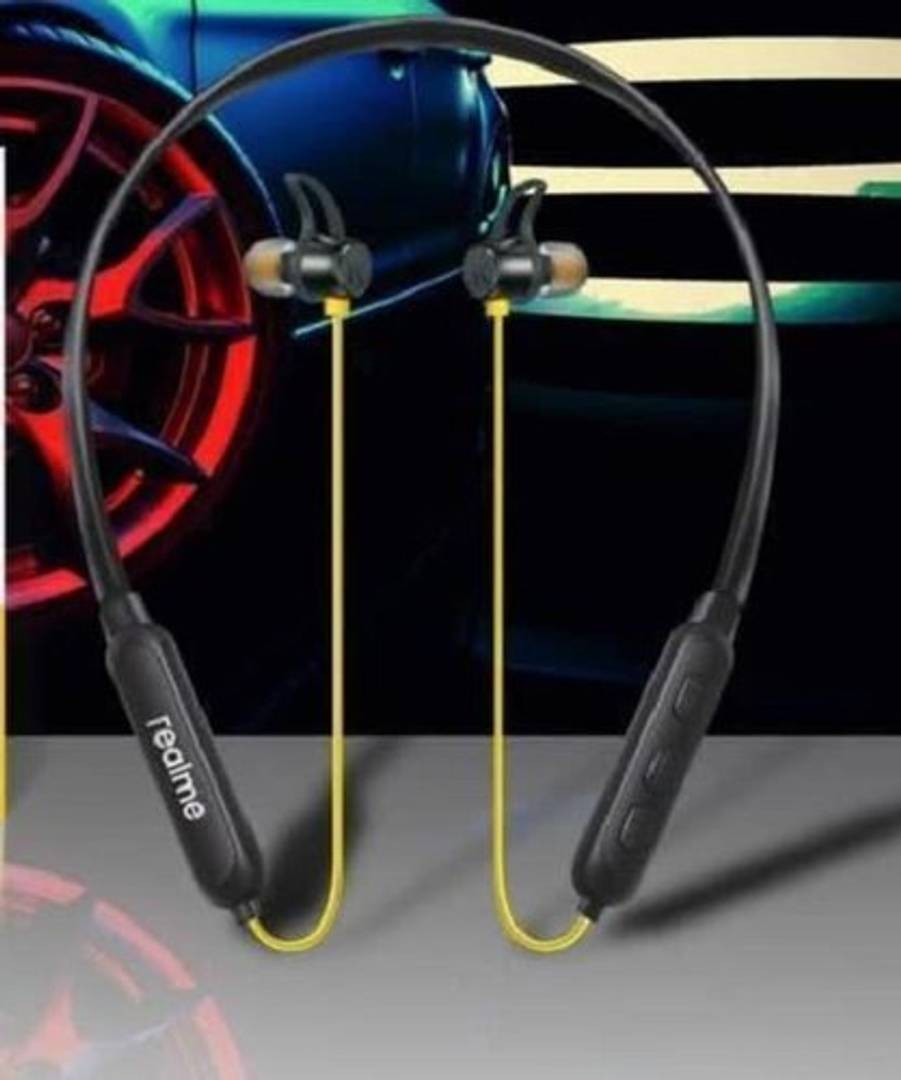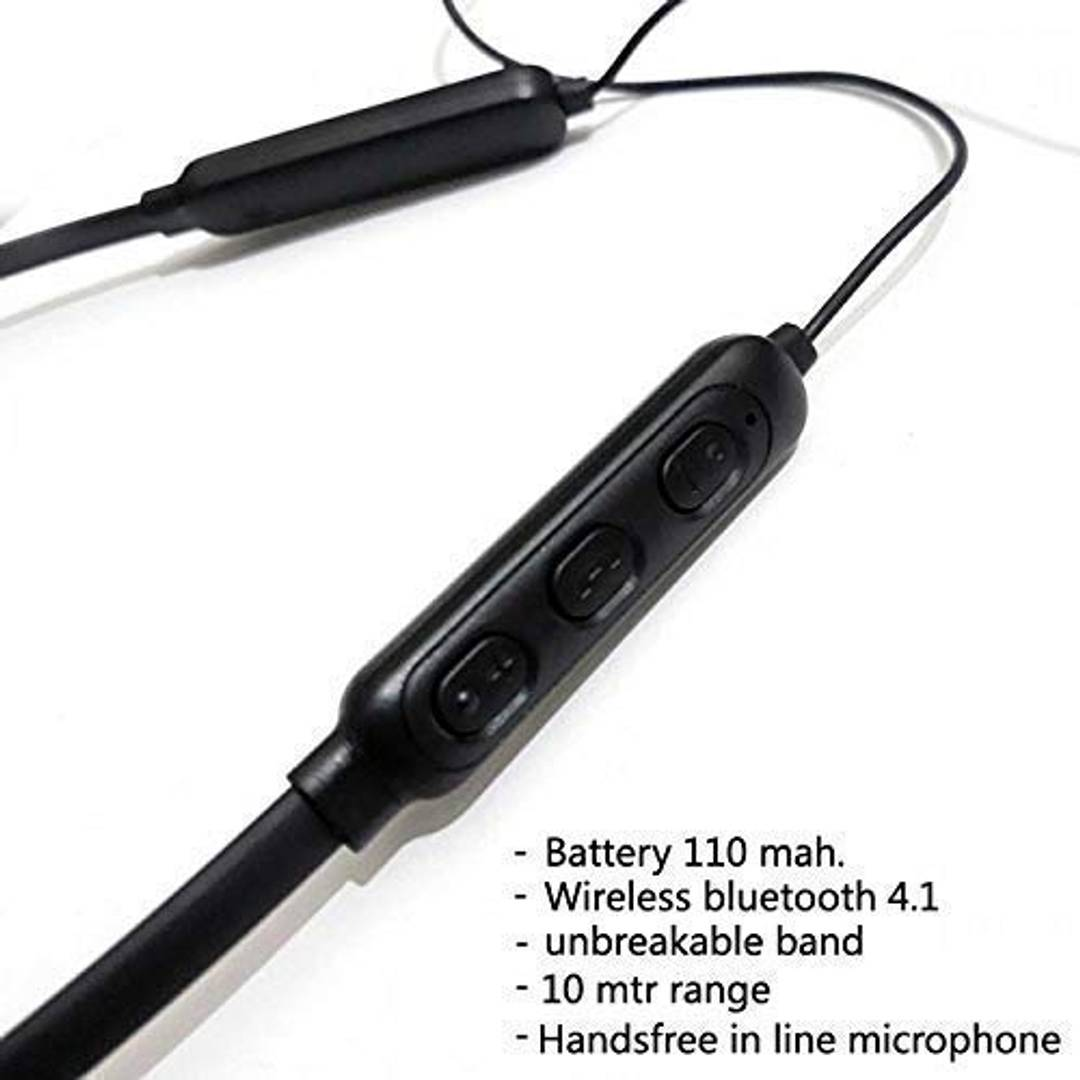Rs. 899.00
Rs. 1,799.00Rs. 721.00
Rs. 1,499.00
Sold Out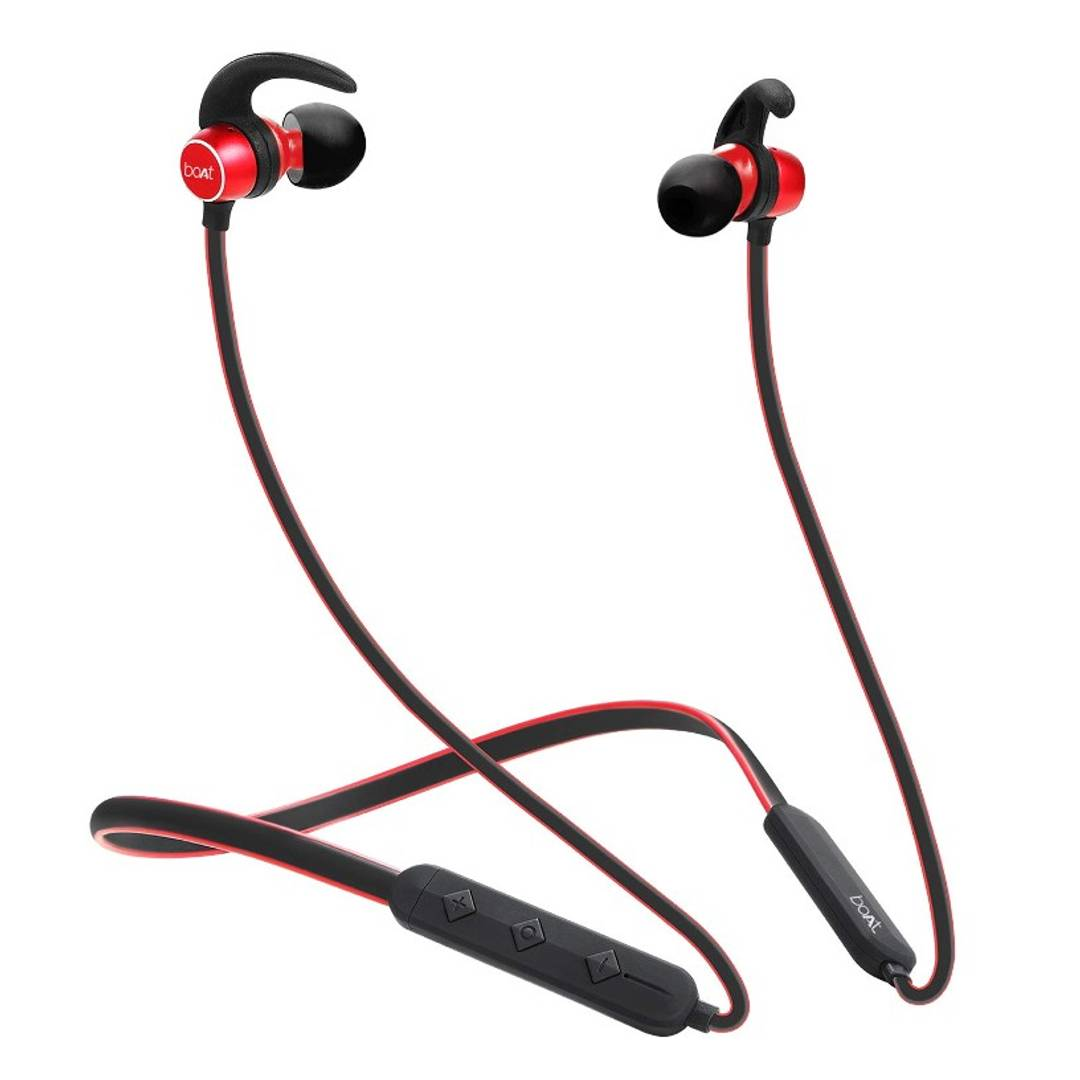Rs. 749.00
Rs. 1,599.00
Sold OutRs. 549.00
Rs. 1,499.00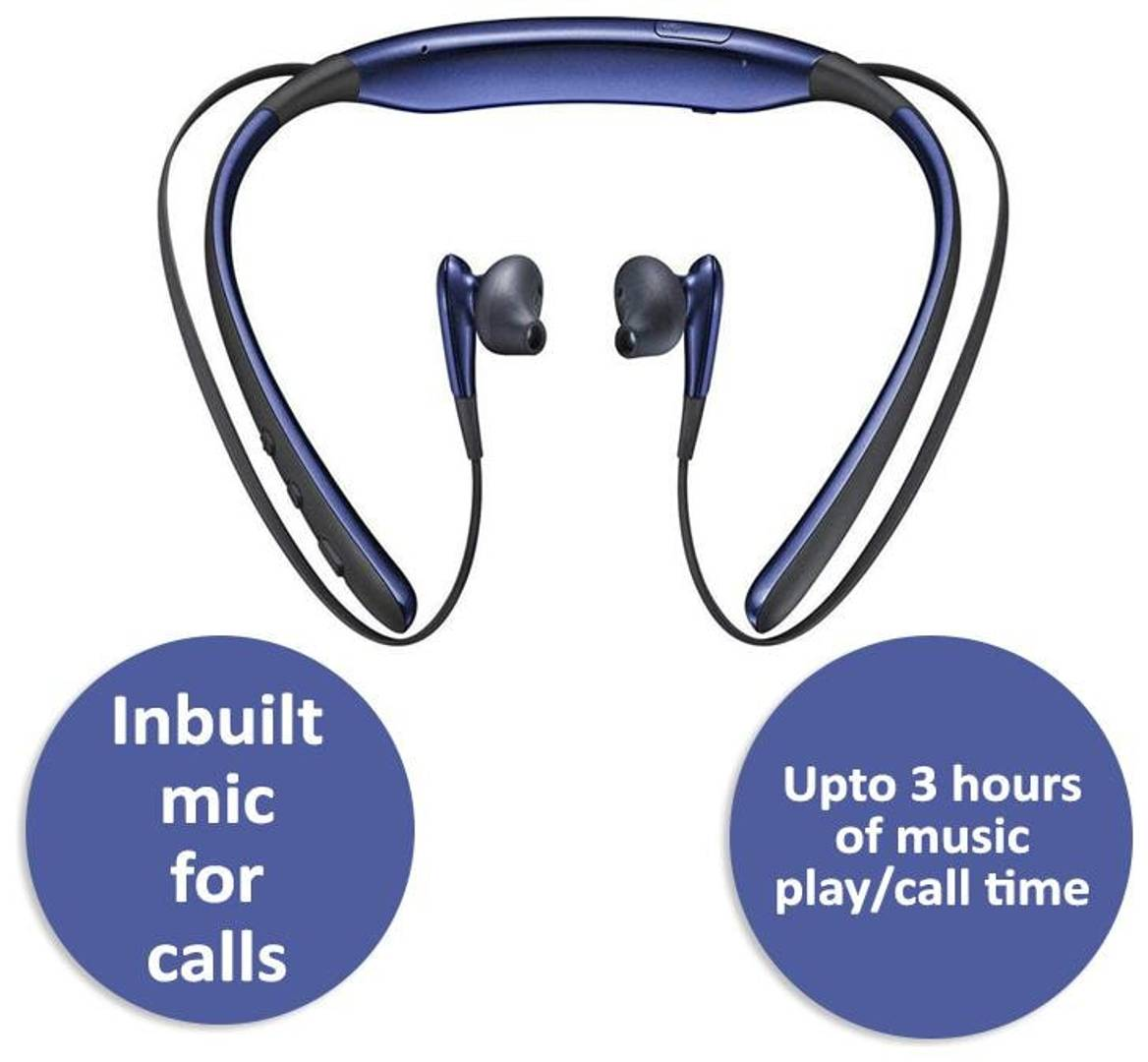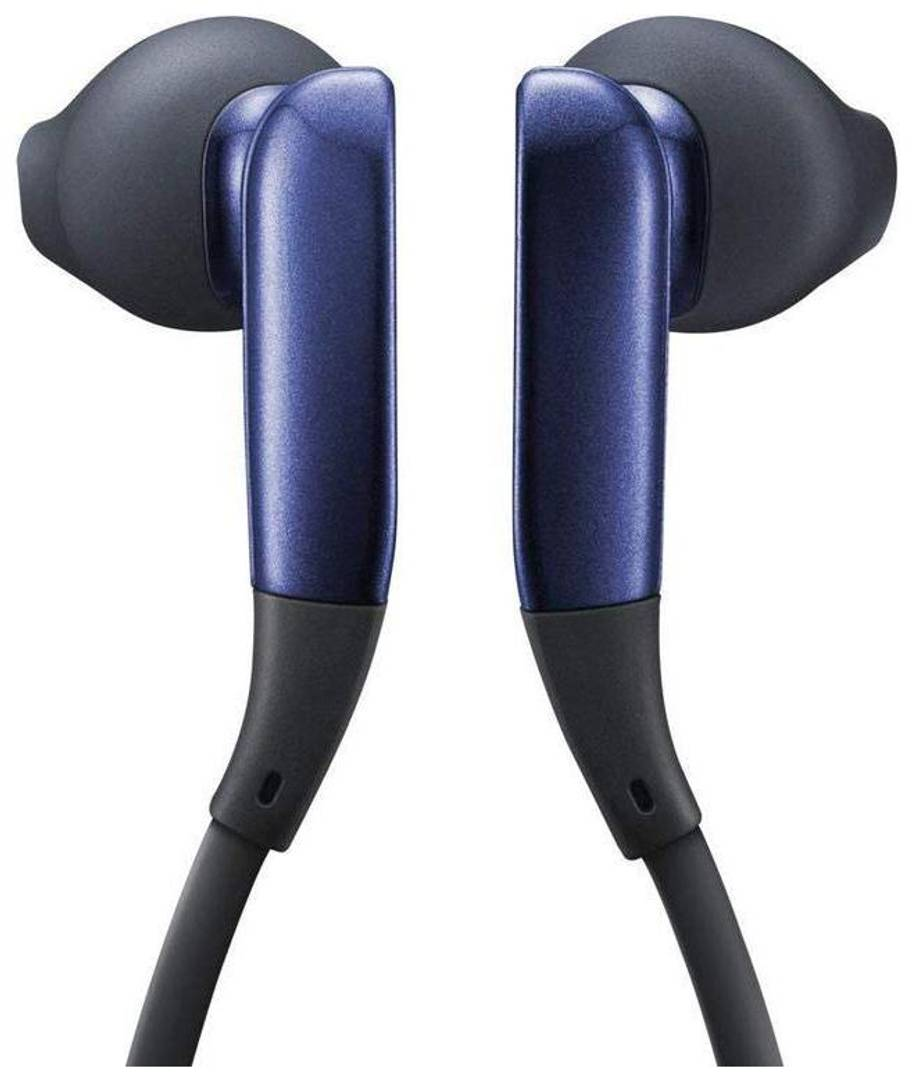Rs. 1,000.00
Rs. 1,899.00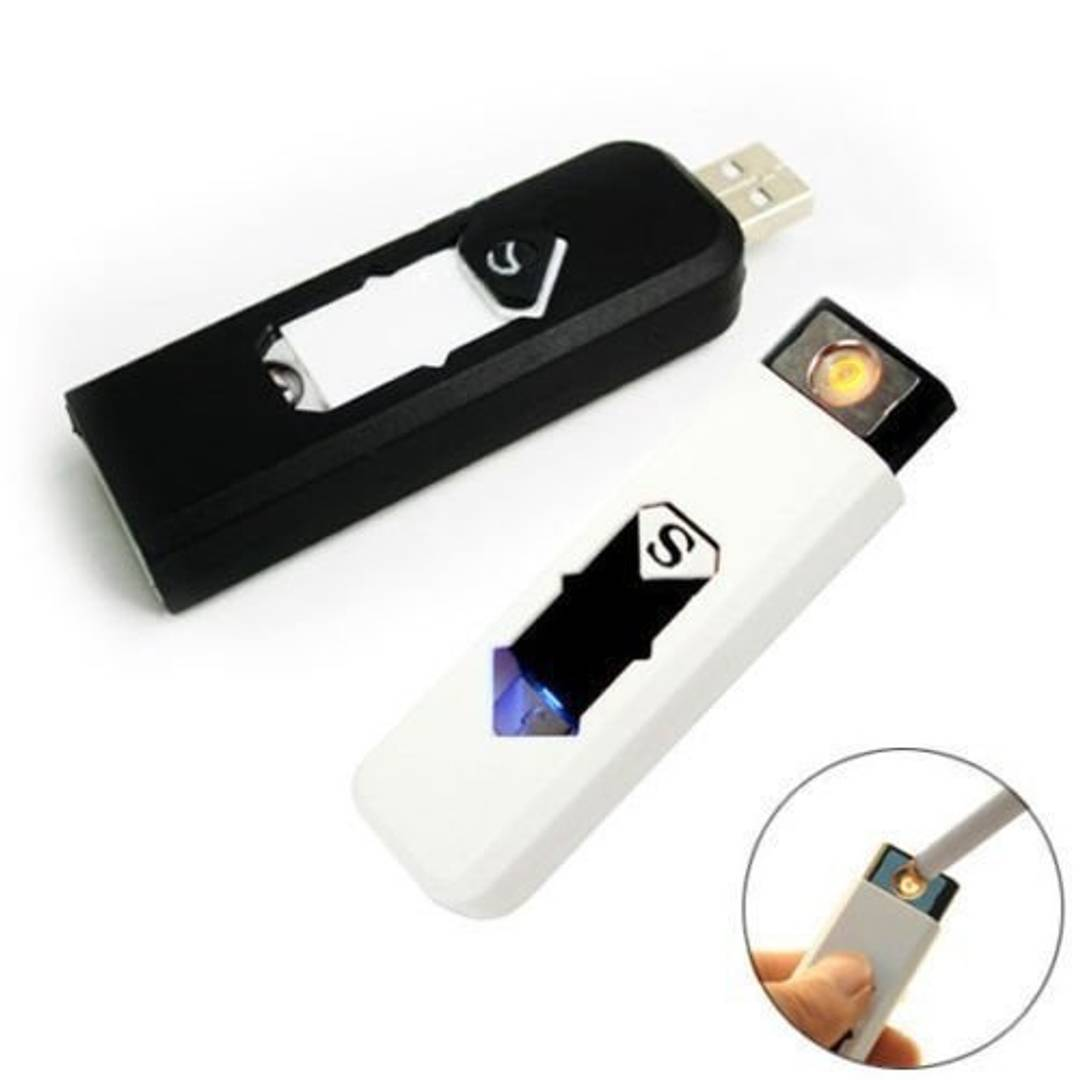Rs. 749.00
Rs. 1,499.00
Sold OutRs. 599.00
Rs. 999.00Rs. 1,959.00
Rs. 2,875.00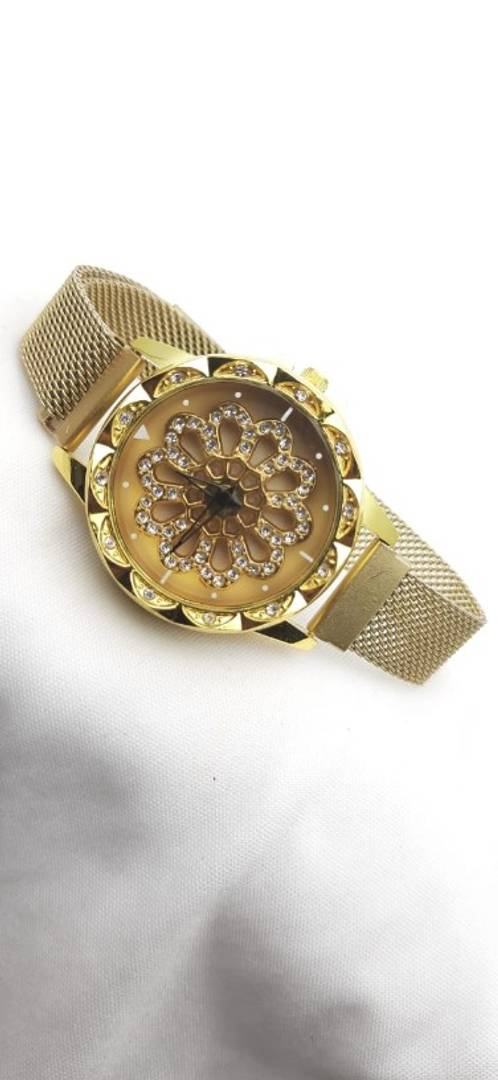Rs. 649.00
Rs. 1,299.00
Sold OutRs. 499.00
Rs. 1,499.00

No reviews yet
0%
(0)
0%
(0)
0%
(0)
0%
(0)
0%
(0)